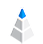# Mean vs. Median

The term “average” can often mislead us and hide valuable and reliable information from our data. It is hard to decide which number we need to use.

In everyday statistics and news or even in scientific research, the result is often presented as the mean value. The median, however, gives you much better and more reliable information about your database.

## The Difference Between Mean and Median

The mean is the average you already know: just add up all the numbers, then divide by the number of numbers. The median is the middle value in a list of numbers. To find the median, you need to list the numbers in numerical order first.

To see the difference, here is an example made from our own Customer Behavior in the Banking Sector dataset, to illustrate the mean and median.On the first chart you can see the duration of contacts made with customers in seconds. The middle 80% range here is 59 to 551 seconds. It is necessary to see this range because outlier values in our data can distort the results and visualizations.

The green line shows the median (179 seconds) and the blue line shows the mean value (210.44 seconds). If we want to know the average time spent on customer contacts, the mean and the median show us very different information. So, which one should we trust?

We recommend you to choose the median instead of the mean. Below you can read the reasons.

## The Outlier Problem

On the next chart, you can see the same dataset but visualized in full range, including outliers (0 to 4920 seconds). That is a big range. It is also easy to see the difference between the median (180 seconds) and the mean (258,29 seconds) values, but now we can also clearly understand how far the outlier values lie.## The Changing Mean

What if we do not involve the outlier? Let’s see what happens when we exclude more and more outlier values. Using a filter we can see values on the third chart from 0 to 3200 now.The value of the mean will change (decrease), but the median will not until a bigger change occurs.

Therefore, the median is a more reliable and more stable number than the mean.

Important to notice, that the outlier value will not throw off the result.

## Standard Deviation

Standard deviation is often used to support the understanding of the average. It helps to describe our results by not using one number only, but it is not understandable to everybody. Instead, it is better to use the midrange.

For this, we can use the IQR (interquartile range), which can show us the range of the middle 50% of the values. However, what about the other 50%? It is better if we check the 10-90 percentile range- the middle 80% range of values. With this number, we can describe the bottom and the top 10% of our data.

## Histograms

It has been proven that people can understand shapes and visualized data better than just plain numbers. Therefore, we recommend using histograms. Below you can see one more reason to use it.You may have a big amount of minimum and maximum values but just a few from the middle. In our case, the mean and the median will be the same number (5), and without a histogram, you will not be able to see the real meaning of your data.## Summary

Using mean value in data science is a risky decision. It can often mislead you and hide the true results of your analysis. If you have outliers in your data, using the mean will distort the information and can give you false insights.

By visualizing your data you can detect outliers, also you can better understand the underlying dataset of yours. Knowing the background of your data can help you to avoid false assumptions.